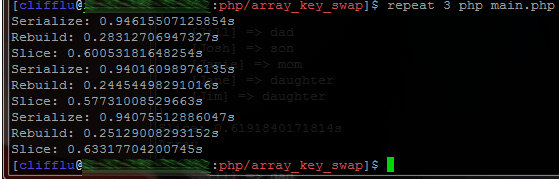PHP 的陣列相當強大，其實作接近有序的 hash table，能以 stack (array_push, array_pop), queue (array_shift, array_unshift), 或 iterator 介面 (foreach) 去操作，並以 \$array[‘key’] = ‘value’ 的方法賦值。

1. 排序：依照特定原則重新排序整個陣列 / hash table
2. 反序與亂序：array_reverse, shuffle
3. 部份替代：array_splice (若為 numeric key 則不保存，亦即會被設為該鍵值組的實際序數）
4. 重建：array_unique, …

PHP 提供 serialize / unserialize （序列化/去序列化）函數，可將任何物件（class 要先能載入定義）與文字間做轉換；而陣列對文字的轉換規則相當單純。因此我認為，將原陣列經過 serialize、替換、unserialize 之後，除了能得出正確答案之外，速度應該會比較快。* 同場加映：

 array_splice array_slice 功能 將陣列中的特定範圍移除並取代 取出陣列中的特定範圍 回傳值 被取代的範圍內容 被取出的範圍內容 傳入陣列形式 passing by reference, 且會被更動 passing by value, 不影響呼叫端的值``` \$value) {
if (\$key === \$key1) {
\$ret[\$key2] = \$array[\$key1];
} else if (\$key === \$key2) {
\$ret[\$key1] = \$array[\$key2];
} else {
\$ret[\$key] = \$value;
}
}
return \$ret;
}

// ===================================
// Array_Splice
// ===================================

function skn_slice(\$array, \$k1, \$k2) {
\$keys = array_keys(\$array);

\$k1p = array_search(\$k1, \$keys);
\$k2p = array_search(\$k2, \$keys);

\$is_k1_larger = \$k1p > \$k2p ;

\$kminp = \$is_k1_larger ? \$k2p : \$k1p;
\$kmaxp = \$is_k1_larger ? \$k1p : \$k2p;

\$kmin = \$keys[\$kminp];
\$kmax = \$keys[\$kmaxp];

\$vmin = \$array[\$kmin];
\$vmax = \$array[\$kmax];

\$array_s1 = array_slice(\$array, 0, \$kminp);
\$array_s2 = array_slice(\$array, \$kminp + 1, \$kmaxp);
\$array_s3 = array_slice(\$array, \$kmaxp + 1);

\$array = \$array_s1 + array(\$kmax => \$vmin) + \$array_s2 + array(\$kmin => \$vmax) + \$array_s3;

// 使用 array_merge 會多花約 20% 的執行時間，猜想與 \$array[] 效能高過 array_push(\$array, ...)
// 原因相同：函式調用的成本。
// 同理，若改成呼叫 array_splice 兩次（而非 array_slice 三次）可能可以降低執行時間。

// \$array = array_merge(
//     \$array_s1, array(\$kmax => \$vmin),
//     \$array_s2, array(\$kmin => \$vmax),
//     \$array_s3);

return \$array;
}

// ===================================
// Main
// ===================================

\$their_name = array(
//       Key   =>  Value
'Josh'  => 'son',
'Jamie' => 'mom',
'Jane'  => 'daughter',
'Jill'  => 'daughter'
);
\$k1 = 'Jim';
\$k2 = 'Jill';

\$times = 10000;
\$cnds = array(
'Serialize',
'Rebuild',
'Slice',
);

foreach(\$cnds as \$cnd) {
\$fp_main = "skn_".strtolower(\$cnd);

if (!function_exists(\$fp_main))
continue;

\$ts = microtime(true);
for(\$i=0;\$i<\$times;\$i++)
\$output = \$fp_main(\$their_name, \$k1, \$k2);

echo "\$cnd: ". (microtime(true) - \$ts) . "s\n";
print_r(\$output);
}
?>
```